Courses

# Operating Principles And Construction Of Single Phase Transformers Electrical Engineering (EE) Notes | EduRev

## Electrical Engineering (EE) : Operating Principles And Construction Of Single Phase Transformers Electrical Engineering (EE) Notes | EduRev

The document Operating Principles And Construction Of Single Phase Transformers Electrical Engineering (EE) Notes | EduRev is a part of the Electrical Engineering (EE) Course Electrical Machines.
All you need of Electrical Engineering (EE) at this link: Electrical Engineering (EE)

Operating Principles and Construction of Single Phase Transformers

In the last chapter we have shown that in alternating current circuits the voltage level can be easily stepped up or stepped down using a transformer. The transformer we have shown is a set of coils that are magnetically coupled; in order to couple a set of coils we make the following arrangement.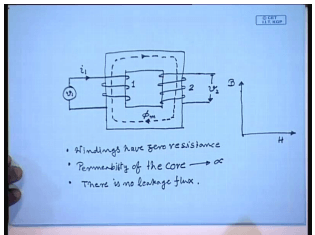We choose a core made up of ferromagnetic material and put winding on them. So, if we connect a voltage source v 1 here, there will be a current flowing into the coil i 1, and this will generate a voltage v 2 in the second coil by the principle of induction that is Faraday’s law. The current in the first coil produces a flux that circulates through the core and links the second coil. Since alternating circuits the flux is also alternating, there is a rate of change of flux linkage in the second coil as a result of which a voltage v 2 is generated, but this is the basic operating principle of a transformer. We can get good insight into the operation of this transformer, if we make certain simplifying assumption. For example, the first assumption we make that the windings are lossless; windings are lossless that is they have 0 resistance, of course that is an ideal winding.

The second assumption is regarding the core we assume that the permeability of the core is infinity which means in order to establish any finite amount of flux the current drawn will be 0. This implies the permeability of the core infinity implies the B H curve of the material is the y axis; that is for any finite B the corresponding H is 0; therefore, the required ampere turns is 0, and hence the current is 0. As a corollary of this second assumption, since the permeability of the core is infinity there will also be no leakage in this construction; that is all the flux produced by coil one will be confined inside the core, and there will be no flux that will be flowing through the air. Since all the flux will be flowing through the core all the flux will also leave coil two.

So, the third assumption which is not really a new one, but a consequence of the second assumption is that there is no leakage flux. So, the transformer for which these assumptions are made is called an ideal transformer. Now this ideal transformer is a conceptual device; you can understand that no such practical transformer exist for which all these three assumptions hold. However, the introduction of an ideal transformer as a conceptual device does help in understanding how a practical transformer works, and we will later on relax this assumption and try to arrive at the model of a practical single phase transformer. But for the time being let us spend some time to find out what are the consequences of these three assumptions, and how we can analyze a single phase ideal transformer.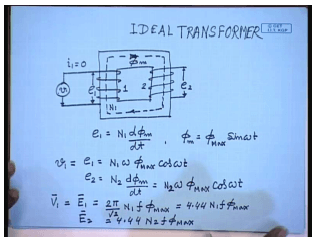As we have mentioned when a voltage v 1 is applied across coil one of an ideal transformer a flux phi m is established in the core; however, in order to just establish this flux no current is drawn from the source; that is under this condition i 1 is identically equal to 0. Then we should also conclude that the voltage induced across coil one e 1 must exactly balance the applied voltage v 1, so that the current is 0. Now by Faraday’s law e 1 is given by N 1 d phi m d t where N 1 is the number of turns of coil one. Let us assume that phi m in steady state is given by the expression phi max sin omega t then e 1 is equal to N 1 omega phi max cosine omega t.

Similarly, the induced voltage e 2 in coil two will be given by e 2 equal to N 2 d phi m d t. It is to be noted since in ideal transformer there is no leakage the total flux linking the coil one and coil two are same. Both are given by phi max sin omega t. If I write in phasor notation that is I want to find out the r. m. s value of e 1. So, v 1 equal to E 1 equal to 2 pi by root 2 N 1 f phi max. This is the well known formula 4.44 N 1 f phi max, and v 2 similarly e 2 equal to 4.44 into N 2 f phi max.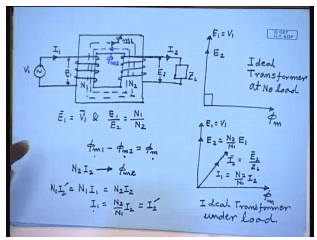Therefore what we can say about an ideal transformer? If we have an ideal transformer where we apply a voltage v 1 across its first coil with number of turns N 1 then the induced voltage on the second coil with turns N 2; it is related to the voltage induced in the first coil by the following relationship. E 1 equal to v 1 and E 1 by E 2 equal to N 1 by N 2; not only that E 1 and v 1 are in phase, e 2 is also in phase with e 1.

The phasor diagram can be drawn as follows. The previous calculation we have shown if the flux form is given by the time function phi max sine omega t then the induced voltage is given by the function N 1 omega phi max cos omega t. Now cos omega t leads sine omega t by an angle of 90 degree; therefore, you can draw the phasor diagram as follows. Taking the flux to be the reference phasor the voltage is v 1 e 1 and e 2 leads it by 90 degree. So, this is the phasor diagram of an ideal transformer at no load; no load since no load has been connected on the secondary side.
Now suppose I connect a load on the secondary side of impedance Z L; obviously, there is an induced voltage here current I 2 will flow which will be determined by the magnitude and phase angle of the impedance Z L. However, as soon as the current I 2 starts flowing in the second coil, by Faraday’s law it will try to oppose the very cause which is due; that is it will try to send a flux which is opposite to the flux established by coil one as a result of which the total flux linking coil one will become phi m minus phi m 2. So, the total flux linking coil one will reduce, hence e 1 will reduce; however, since there is no impedance between the applied voltage and the coil there will be current drawn from the source v 1 of such magnitude so that the induced voltage across coil one always balances the applied voltage v 1.

Otherwise this current will keep on increasing which means the flux produced by coil one will now change to phi m 1, and in steady state phi m 1 minus phi m 2 will still be equal to phi m so that the induced voltage e 1 and e 2 does not change. So, what will be the magnitude of the current I 1? Obviously, if phi m 1 minus phi m 2 is same as phi m then phi m 2 is produced due to an ampere term of N 2 I 2 the ampere terms N 2 I 2 produces the flux phi m 2; therefore, this ampere turn must be cancelled by the ampere turns of the primary coil so that the net flux still remains at phi m. So, this should be equal to. So, the current drawn from the source must produce a cancelling ampere turns N 1 I 1, so that the flux produced in the core remains constant at phi m.

Therefore, we can say the N 1 I 1 should be equal to N 2 I 2 or I 1 equal to N 2 by N 1 I 2. This is sometimes called the reflected load current I 2 dash; therefore, when we put a load on the secondary of an ideal transformer a reflection current I 2 dash flows in the primary side so which completely cancels the ampere turns of the secondary side. The relationship is N 1 I 2 dash is equal to N 1 I 1 equal to N 2 I 2. Therefore we have the following relation in the phasor; again taking the net flux linkage phi m to be the reference phasor we have seen that under load condition E 1 and E 2 remains unchanged; that is E 1 still is equal to v 1, and E 2 is given by E 1 by E 2 is equal to N 1 by N 2. The current I 2 is determined by the loading Z L; the current I 1 will be in phase with I 2, its magnitude will be I 1 equal to N 2 by N 1 I 2. This is the phasor diagram of the ideal transformer under loaded condition.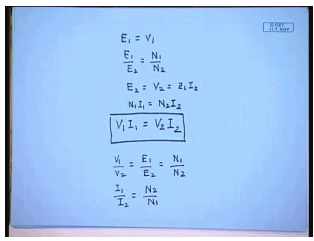So, for an ideal transformer we have seen E 1 equal to v 1, E 1 by E 2 equal to N 1 by N 2, E 2 equal to v 2 equal to Z L I 2, and N 1 I 1 equal to N 2 I 2. In other words from this relation we can show v 1 I 1 equal to v 2 I 2; that is in ideal transformer the pure energy convertor whose input v a is always equal to the output v a, and the voltage and current are transformed by the terms ratio that is v 1 by v 2 equal to E 1 by E 2 equal to N 1 by N 2. Similarly, I 1 by I 2 equal to N 2 by N 1; this relation holds for an ideal transformer. As we have mentioned an ideal transformer is a pure energy convertor, and it is a conceptual device; it cannot be made in practice. So, a practical single phase transformer is an approximation of an ideal transformer, where are the differences? We had made three assumptions while formulating the idea of an ideal transformer.

That is we said that the windings are lossless; no practical winding will be lossless. There will be some resistance in every winding. How do we? Similarly the core of a practical transformer will not have infinite permeability. It will have very high permeability but not infinite; therefore, even under no load condition a practical single phase transformer will draw some current to establish the flux in the core. This is a called the magnetization current. Then third as a result of finite permeability of the core material there will invariably be some leakage flux in the single phase practical transformer the leakage flux will circulate through the air, and it will link only the coil that produces that flux and not the other coil.
We will shortly see how to incorporate this into the model of a practical single phase transformer, and how to develop a model of a practical single phase transformer incorporating the ideal transformer, but before that let us turn our attention to some of the constructional details of a practical single phase transformer.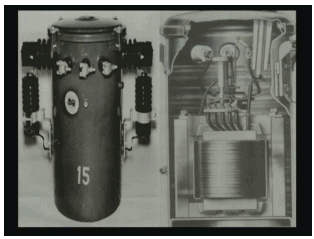The figure on your screen shows the left hand side shows the outward appearance of a practical single phase transformer. This drum, this can like appearance is due to the casing. The actual transformer is inside the casing; the actual transformer is inside this casing. These protruding parts are basically insulators through which external connection is made to the transformer inside. Now if this is cut open, inside we will find the actual transformer which is this object.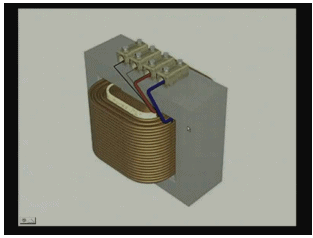Let us have a closer look of how this looks like. If we take a closer look the practical transformer consists of an iron core this member on which a set of windings are placed. So, the main parts are core and set of windings.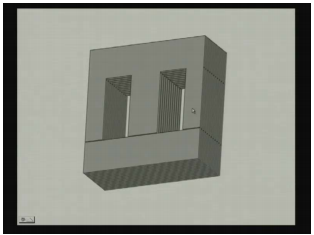Taking a closer look at the core we will find that this core is not a solid block of iron; rather it is made of a large number of steel plates of steel sheets which are cut in a particular fashion. Here it is shown that these steel sheets are cut in E and I shapes; then many such E and I steel sheets are placed together to form the core. Now this is done; these are called transformer laminations. The transformers are laminated in order to reduce the eddy current loss.
Now, you are all familiar with the mechanism of eddy current loss. If there is a coil which produces an alternating flux the flux flows through the core different parts of the core. Since this is an alternating flux it produces circulating current around them; iron being a conducting material a finite amount of current flows through the core, and it causes loss. By laminating we tend to increase the resistance of the circulating current path so that the loss is reduced. This is called laminating the transformer core almost all practical or frequency transformers are laminated.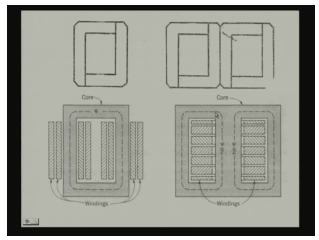Now this shows the different types of lamination that can be used. Now here we should say that this is just one shape of the core. There are basically two types of core used that are used with single phase transformer; one is called the core type core, another is the shell type. Difference is here the windings are placed on the core; that is the windings around the core in the shell type construction it is rather the core that surrounds the winding. So, these are the two different construction of the core; one is the core type and other is the shell type. In both cases in order to have good coupling between the primary or the primary and the secondary order the H v and L v winding; they are both made into different sections and in terming hold. Here you can see that both the winding are divided into two parts and placed on both the links of the core. In the shell type construction each of the windings the primary and the secondary are divided into different sections, and then they are alternately placed, so that the coupling between the total winding is good, and the leakage is kept to a minimum.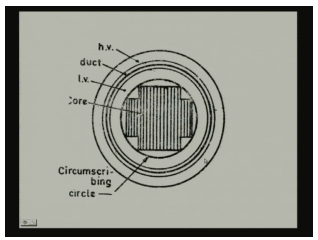If we look at the cross-section of the central line then this is what we will find. The inside H portion shows the transformer laminations; on the lamination usually a cylinder will be placed which is made up of insulating material so that it provides insulation between the core and the winding that will be placed next. The low voltage winding is placed on that insulating cylinder, and then once all complete low voltage winding is placed then there will be another cylinder which will enclose the low voltage winding, and on top of which the high voltage winding will be placed by keeping a gap between the low voltage and the high voltage winding which provides for the duct for the cooling medium.

The transformers while operation carry current, and hence there will be I square or R loss in both the windings that heat needs to be taken out of the transformer so that the temperature rise of the transformers can be maintained. Therefore, paths must be provided for the cooling medium usually oil to flow through the core and the windings; it is the purpose of the duct in between. So, the core as we can see has a strange set like a cross. This is called the cruciform core; the reason is simple. The l. v. and h. v. winding both are circular, and they are placed on cylindrical surfaces.
The purpose of making this a cruciform rather than a square or a rectangle is to be able to utilize the most of the area inside the cylinder for very small transformers such as these even rectangular or square shape cores are used, but as the transformer rating goes higher and higher, higher number of steps are used so that the periphery of the transformer core approximates a circle, and the area inside the winding cross-section is fully utilized.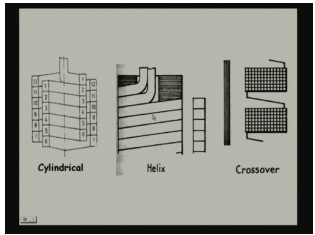Now coming to the windings, there are many different types of windings that are used with transformers depending on their voltage and current rating. For single phase transformer usually three types of windings are used. For the low voltage winding for a given cable you can understand the low voltage winding; voltage rating is less, but the current rating is large for which normally two types of winding either a cylindrical multilayer or a helical winding is used, while for the high voltage either a cylindrical or a crossover winding is used. This picture shows the diagram of different winding arrangement. The low voltage winding are usually made up of rectangular conductors may be a single conductor or a large number of strips taken together and found on the insulating cylinder of the low voltage winding.
Between turns another set of duct and insulation will be provided on which a second layer can be found. For the high voltage winding, high voltage winding will usually have a large number of turns, but its current rating will be small. There can be steel made up of rectangular wires or even circular wires. In the crossover winding generally high voltage windings are divided into several groups, and the groups are found separately, and then they are connected in series to form the high voltage winding. Each group can have many numbers of turns, and there can be arranged in multiple layers.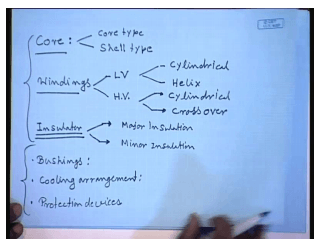Therefore to summarize the constructional details of a single phase transformer we can say that the main parts of a single phase transformer are the core. This is usually made of silicon steel and can be either hot rolled or cold rolled; for large rated transformers normally cold roll oriented or shear Geo steel is used. For smaller transformer hot rolled steel is used; this is silicon steel. The purpose of the core is to provide the path for flux, high permeability path for the magnetic flux, and the cores are of two types. The transformer core can be of core type or shell type. The next important part of a transformer is the windings; there are two windings in a transformer.
Normally the two winding transformer there will be two windings; one is the L V winding, another is the H V winding. The windings are made up of aluminum or copper conductors either round or square cross-section. Square cross-section conductors can be made up of solid conductor or multiple strips connected in parallel. The L V windings are usually of two types; one is the cylindrical or helix. The H V winding can also be of two type either cylindrical or crossover. The next important constructional feature is the insulator. Insulators are used in different places of a transformer, and they are classified as major insulation which are between let us say core and the L V winding, L V winding and the H V winding, and the minor insulation which are usually between the turns of the same winding or the layers of the same winding.

Now there are different types of insulation materials that are used. For example, pressboard cylinders, then paper, transformer oil at different purpose, different insulations are used. The insulation material are characterized by the maximum temperature at which they can maintain their insulation properties, and this is the weakest constructional component of a transformer, and more often not the quality of the insulator will determine the reliability or even the size of a transformer. Other than these three main constructional elements, there are many other elements that are used; for example, the bushings. The bushings are these protruding elements through which external connection is made to the transformer. These are usually porcelain cylinders hollow porcelain cylinders which these sets through which the connecting conductors are placed. They can be for high voltage, they can be oil field, or they can be of capacitor type.

So, bushing is provided for external connection and then cooling arrangement. For small transformer separate cooling arrangements are not provided. The cooling is provided by the tank itself through which the transformer is placed. For large transformer they are usually immersed in transformer oil so that heat can be extracted from the inner parts of the transformer that is the core, and the L V winding which is inside the H V winding. For even larger transformer the external cooling pipes or kings may be provided for even larger three phase transformer forced cooling by oil and with fan cooling can be provided. So, a large number of cooling arrangements are possible, and for depending on the power rating of the transformer a suitable cooling method is chosen, and then we will find the protection arrangements which I will find in the form of for oil field transformer, for conservators, and then explosion vents, Buchholz relay and then lightening arresters and different circuit breakers and relays.
So, these are the essential constructional elements of a single phase transformer that we will normally find. Now coming to the comparison with an ideal transformer there are as we said that for conceptualizing an ideal transformer we made three assumptions; that is the windings are lossless, the permeability of the core material is infinity, and there is no leakage. Now none of these three assumptions hold good for a practical single phase transformer. So, we need to relax these assumptions one by one and arrive at a model of a practical transformer. So, let us see.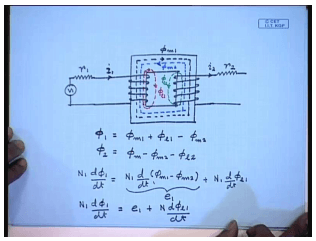Let this be the ideal transformer. It is easy to account for the resistance of the practical transformer coils. They will appear as a lump resistance in series with both the coils; let us call it r 1 and the resistance on the other side r 2. Here the resistances of coil one with turns N 1 and coil two with turn N 2 respectively; however, it is more difficult to relax the condition of the infinite permeable. We said due to infinite permeability in ideal transformer all the flux produced by the coil remains inside the core. So, in practical in an ideal transformer let this be the flux phi m 1 produced by current I 1, and this is the flux phi m 2 produced by current I 2. We have seen that phi m 1 minus phi m 2 remains constant if the applied voltage on coil one is constant.

However, in a practical single phase transformer since the core is not infinitely permeable there will always be some flux which will not flow entirely through the core but part of it will flow through the air. This is called the leakage flux, call it phi l 1. Similarly there will be the leakage flux produced by, oh, current flowing in the second coil phi l 2 which will link only coil two and not coil one. So, what is the total flux linking coil one? Phi 1 equal to phi m 1 plus phi l 1 minus phi m 2; similarly, the total flux linking coil 2 phi 2 is equal to. Therefore, the voltage induced in coil 1 is N 1 d phi 1 d t equal to N 1 d d t of phi m 1 minus phi N 2 plus N 1 d d t of phi l 1. Keeping the analogy with our discussion with the ideal transformer let us call this term to be the induced voltage v 1 when N 1 d phi 1 d t equal to e 1 plus N 1 d phi e 1 d t.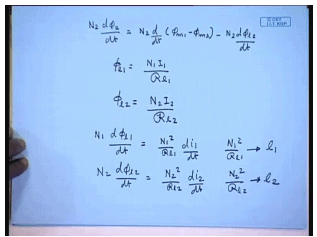Similar equation can be written for the other coil N 2 d phi 2 d t equal to N 2 d d t of phi m 1 minus phi m 2 minus N 2 d phi l 2 d t. Now phi l 1 equal to N 1 I 1 divided by the reluctance of the leakage path r l 1. Similarly phi l 2 equal to N 2 I 2 by the reluctance of the second leakage path R l 2. This can be written as therefore N 1 d phi l 1 d t equal to N 1 square by R L 1 d i 1 d t, and N 2 d phi l 2 d t equal to N 2 square by r l 2 d i 2 d t. The term N 1 square by R L 1 is called the leakage inductance of coil one l 1, and N 2 square r l 2 is called the leakage inductance in l 2.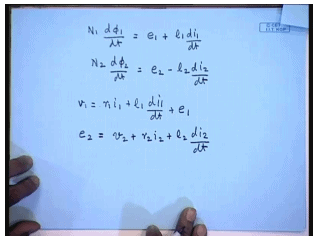Therefore N 1 d phi 1 d t equal to e 1 plus l 1 d i 1 d t and N 2 d phi 2 d t equal to e 2 minus l 2 d i 2 d t. Calling the applied voltage v 1 and the terminal voltage v 2, then we can finally write v 1 equal to r 1 i 1 plus l 1 i 1 d t plus e 1, whereas e 2 equal to v 2 plus r 2 i 2 plus l 2 i 2. This set of equations gives the model of a single phase transformer.

Offer running on EduRev: Apply code STAYHOME200 to get INR 200 off on our premium plan EduRev Infinity!

61 docs|20 tests

,

,

,

,

,

,

,

,

,

,

,

,

,

,

,

,

,

,

,

,

,

;